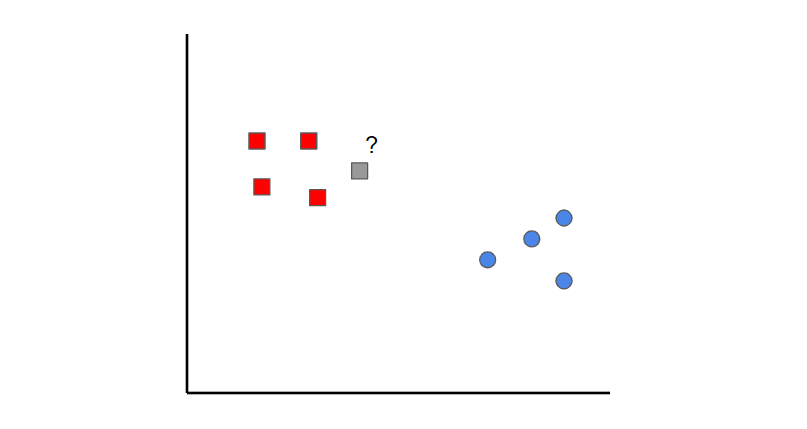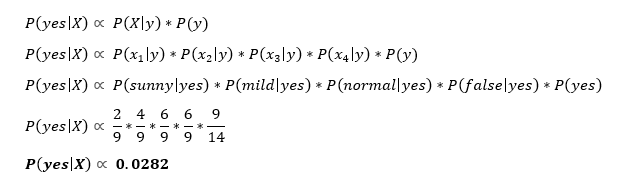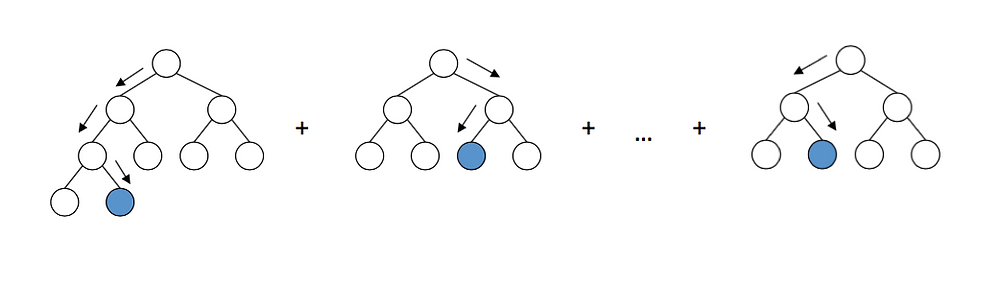Search

# Popular Machine Learning Algorithms You Should Know in 2020

Written by Sergio Antonio BianchiAs my knowledge in machine learning grows, so does the number of machine learning algorithms! This article will cover machine learning algorithms that are commonly used in the data science community.

Keep in mind that I’ll be elaborating on some algorithms more than others simply because this article would be as long as a book if I thoroughly explained every algorithm! I’m also going to try to minimize the amount of math in this article because I know it can be pretty daunting for those who aren’t mathematically savvy. Instead, I’ll try to give a concise summary of each and point out some of the key features.

With that in mind, I’m going to start with some of the more fundamental algorithms and then dive into some newer algorithms like CatBoost, Gradient Boost, and XGBoost.

## Linear Regression

Linear Regression is one of the most fundamental algorithms used to model relationships between a dependent variable and one or more independent variables. In simpler terms, it involves finding the ‘line of best fit’ that represents two or more variables.

The line of best fit is found by minimizing the squared distances between the points and the line of best fit — this is known as minimizing the sum of squared residuals. A residual is simply equal to the predicted value minus the actual value.In case it doesn’t make sense yet, consider the image above. Comparing the green line of best fit to the red line, notice how the vertical lines (the residuals) are much bigger for the green line than the red line. This makes sense because the green line is so far away from the points that it isn’t a good representation of the data at all!

If you want to learn more about the math behind linear regression, I would start off with Brilliant’s explanation.

## Logistic Regression

Logistic regression is similar to linear regression but is used to model the probability of a discrete number of outcomes, typically two. At a glance, logistic regression sounds much more complicated than linear regression, but really only has one extra step.First, you calculate a score using an equation similar to the equation for the line of best fit for linear regression.The extra step is feeding the score that you previously calculated in the sigmoid function below so that you get a probability in return. This probability can then be converted to a binary output, either 1 or 0.To find the weights of the initial equation to calculate the score, methods like gradient descent or maximum likelihood are used. Since it’s beyond the scope of this article, I won’t go into much more detail, but now you know how it works!

## K-Nearest NeighborsK-nearest neighbors is a simple idea. First, you start off with data that is already classified (i.e. the red and blue data points). Then when you add a new data point, you classify it by looking at the k nearest classified points. Whichever class gets the most votes determines what the new point gets classified as.

In this case, if we set k=1, we can see that the first nearest point to the grey sample is a red data point. Therefore, the point would be classified as red.

Something to keep in mind is that if the value of k is set too low, it can be subject to outliers. On the other hand, if the value of k is set too high then it might overlook classes with only a few samples.

## Naive Bayes

Naive Bayes is a classification algorithm. This means that Naive Bayes is used when the output variable is discrete.

Naive Bayes can seem like a daunting algorithm because it requires preliminary mathematical knowledge in conditional probability and Bayes Theorem, but it’s an extremely simple and ‘naive’ concept, which I’ll do my best to explain with an example:Suppose we have input data on the characteristics of the weather (outlook, temperature, humidity, windy) and whether you played golf or not (i.e. last column).

What Naive Bayes essentially does is compare the proportion between each input variable and the categories in the output variable. This can be shown in the table below.To give an example to help you read this, in the temperature section, it was hot for two days out of the nine days that you played golf (i.e. yes).

In mathematical terms, you can write this as the probability of it being hot GIVEN that you played golf. The mathematical notation is P(hot|yes). This is known as conditional probability and is essential to understand the rest of what I’m about to say.

Once you have this, then you can predict whether you’ll play golf or not for any combination of weather characteristics.

Imagine that we have a new day with the following characteristics:

• Outlook: sunny

• Temperature: mild

• Humidity: normal

• Windy: false

First, we’ll calculate the probability that you will play golf given X, P(yes|X) followed by the probability that you won’t play golf given X, P(no|X).

Using the chart above, we can get the following information:Now we can simply input this information into the following formula:Similarly, you would complete the same sequence of steps for P(no|X).Since P(yes|X) > P(no|X), then you can predict that this person would play golf given that the outlook is sunny, the temperature is mild, the humidity is normal and it’s not windy.

This is the essence of Naive Bayes!

## Support Vector MachinesA Support Vector Machine is a supervised classification technique that can actually get pretty complicated but is pretty intuitive at the most fundamental level. For the sake of this article, we’ll keep it pretty high level.

Let’s assume that there are two classes of data. A support vector machine will find a hyperplane or a boundary between the two classes of data that maximizes the margin between the two classes (see above). There are many planes that can separate the two classes, but only one plane can maximize the margin or distance between the classes.

If you want to get into the math behind support vector machines, check out this series of articles.

## Decision TreeA tree has many analogies in real life and turns out that it has influenced a wide area of machine learning, covering both classification and regression. In decision analysis, a decision tree can be used to visually and explicitly represent decisions and decision making. As the name goes, it uses a tree-like model of decisions. Though a commonly used tool in data mining for deriving a strategy to reach a particular goal, it also widely used in machine learning, which will be the main focus of this article.

How can an algorithm be represented as a tree?

For this let’s consider a very basic example that uses titanic data set for predicting whether a passenger will survive or not. The below model uses 3 features/attributes/columns from the data set, namely sex, age, and sibsp (number of spouses or children along).

A decision tree is drawn upside down with its root at the top. In the image on the left, the bold text in black represents a condition/internal node, based on which the tree splits into branches/ edges. The end of the branch that doesn’t split anymore is the decision/leaf, in this case, whether the passenger died or survived, represented as red and green text respectively.

Although, a real dataset will have a lot more features and this will just be a branch in a much bigger tree, but you can’t ignore the simplicity of this algorithm. The feature importance is clear and relations can be viewed easily. This methodology is more commonly known as the learning decision tree from data and the above tree is called the Classification tree as the target is to classify passengers as survived or died. Regression trees are represented in the same manner, just they predict continuous values like the price of a house. In general, Decision Tree algorithms are referred to as CART or Classification and Regression Trees.

So, what is actually going on in the background? Growing a tree involves deciding on which features to choose and what conditions to use for splitting, along with knowing when to stop. As a tree generally grows arbitrarily, you will need to trim it down for it to look beautiful.

## Random Forest

Before understanding random forests, there are a couple of terms that you’ll need to know:

• Ensemble learning is a method where multiple learning algorithms are used in conjunction. The purpose of doing so is that it allows you to achieve higher predictive performance than if you were to use an individual algorithm by itself.

• Bootstrap sampling is a resampling method that uses random sampling with replacement. It sounds complicated but trust me when I say it’s REALLY simple — read more about it here.

• Bagging when you use the aggregate of the bootstrapped datasets to make a decision — I dedicated an article to this topic so feel free to check it out here if this doesn’t make complete sense.

Now that you understand these terms, let’s dive into it.

Random forests are an ensemble learning technique that builds off of decision trees. Random forests involve creating multiple decision trees using bootstrapped datasets of the original data and randomly selecting a subset of variables at each step of the decision tree. The model then selects the mode of all of the predictions of each decision tree (bagging). What’s the point of this? By relying on a “majority wins” model, it reduces the risk of error from an individual tree.For example, if we created one decision tree, the third one, it would predict 0. But if we relied on the mode of all 4 decision trees, the predicted value would be 1. This is the power of random forests!

AdaBoost, or Adaptive Boost, is also an ensemble algorithm that leverages bagging and boosting methods to develop an enhanced predictor.

AdaBoost is similar to Random Forests in the sense that the predictions are taken from many decision trees. However, there are three main differences that make AdaBoost unique:Example of a stump

1. First, AdaBoost creates a forest of stumps rather than trees. A stump is a tree that is made of only one node and two leaves (like the image above).

2. Second, the stumps that are created are not equally weighted in the final decision (final prediction). Stumps that create more errors will have less say in the final decision.

3. Lastly, the order in which the stumps are made is important, because each stump aims to reduce the errors that the previous stump(s) made.

In essence, AdaBoost takes a more iterative approach in the sense that it seeks to iteratively improve from the mistakes that the previous stump(s) made.It’s no surprise that Gradient Boost is also an ensemble algorithm that uses boosting methods to develop an enhanced predictor. In many ways, Gradient Boost is similar to

AdaBoost, but there are a couple of key differences:

• Unlike AdaBoost which builds stumps, Gradient Boost builds trees with usually 8–32 leaves.

• Gradient Boost views the boosting problem as an optimization problem, where it uses a loss function and tries to minimize the error. This is why it’s called Gradient boost, as it’s inspired by gradient descent.

• Lastly, the trees are used to predict the residuals of the samples (predicted minus actual).

While the last point may have been confusing, all that you need to know is that Gradient Boost starts by building one tree to try to fit the data, and the subsequent trees built after aim to reduce the residuals (error). It does this by concentrating on the areas where the existing learners performed poorly, similar to AdaBoost.

## XGBoostXGBoost is one of the most popular and widely used algorithms today because it is simply so powerful. It is similar to Gradient Boost but has a few extra features that make it that much stronger including…

• A proportional shrinking of leaf nodes (pruning) — used to improve the generalization of the model

• Newton Boosting — provides a direct route to the minima than gradient descent, making it much faster

• An extra randomization parameter — reduces the correlation between trees, ultimately improving the strength of the ensemble

• Unique penalization of trees

For example-I strongly recommend that you watch StatQuest’s video to understand how the algorithm works in greater detail. You can read the full research paper here.

## LightGBM

If you thought XGBoost was the best algorithm out there, think again. LightGBM is another type of boosting algorithm that has shown to be faster and sometimes more accurate than XGBoost.What makes LightGBM different is that it uses a unique technique called Gradient-based One-Side Sampling (GOSS) to filter out the data instances to find a split value. This is different than XGBoost which uses pre-sorted and histogram-based algorithms to find the best split.

Reminds me of THIS-## CatBoost

CatBoost is another algorithm based on Gradient Descent that has a few subtle differences that make it unique:

• CatBoost implements symmetric trees which help in decreasing prediction time and it also has a shallower tree-depth by default (six)

• CatBoost leverages random permutations similar to the way XGBoost has a randomization parameter

• Unlike XGBoost however, CatBoost handles categorical features more elegantly, using concepts like ordered boosting and response codingOverall, what makes CatBoost so powerful is its low latency requirements which translates to it being around eight times faster than XGBoost.

Catboost achieves the best results on thebenchmark-If you made it to the end, congrats! You should now have a better idea of all of the different machine learning algorithms out there.

Don’t feel discouraged if you had a harder time understanding the last few algorithms — not only are they more complex but they’re also relatively new! So stay tuned for more resources that will go into these algorithms in greater depth.

### You can read our previous article on Machine Learning-

(Click on the title to view article)

As always, I wish you the best in your data science and machine learning endeavors. If you liked this article, I’d appreciate it if you share it, hope to see you soon! :)

### Additionally, here is a list of all commonly used Machine Learning algorithms-

1. Regression Algorithms

• Ordinary Least Squares Regression (OLSR)

• Linear Regression

• Logistic Regression

• Stepwise Regression

• Multivariate Adaptive Regression Splines (MARS)

• Locally Estimated Scatterplot Smoothing (LOESS)

2. Instance-based Algorithms

• k-Nearest Neighbour (kNN)

• Learning Vector Quantization (LVQ)

• Self-Organizing Map (SOM)

• Locally Weighted Learning (LWL)

3. Regularization Algorithms

• Ridge Regression

• Least Absolute Shrinkage and Selection Operator (LASSO)

• Elastic Net

• Least-Angle Regression (LARS)

4. Decision Tree Algorithms

• Classification and Regression Tree (CART)

• Iterative Dichotomiser 3 (ID3)

• C4.5 and C5.0 (different versions of a powerful approach)

• Chi-squared Automatic Interaction Detection (CHAID)

• Decision Stump

• M5

• Conditional Decision Trees

5. Bayesian Algorithms

• Naive Bayes

• Gaussian Naive Bayes

• Multinomial Naive Bayes

• Averaged One-Dependence Estimators (AODE)

• Bayesian Belief Network (BBN)

• Bayesian Network (BN)

6. Clustering Algorithms

• k-Means

• k-Medians

• Expectation Maximisation (EM)

• Hierarchical Clustering

7. Association Rule Learning Algorithms

• Apriori algorithm

• Eclat algorithm

8. Artificial Neural Network Algorithms

• Perceptron

• Back-Propagation

• Hopfield Network

• Radial Basis Function Network (RBFN)

9. Deep Learning Algorithms

• Deep Boltzmann Machine (DBM)

• Deep Belief Networks (DBN)

• Convolutional Neural Network (CNN)

• Stacked Auto-Encoders

10. Dimensionality Reduction Algorithms

• Principal Component Analysis (PCA)

• Principal Component Regression (PCR)

• Partial Least Squares Regression (PLSR)

• Sammon Mapping

• Multidimensional Scaling (MDS)

• Projection Pursuit

• Linear Discriminant Analysis (LDA)

• Mixture Discriminant Analysis (MDA)

• Flexible Discriminant Analysis (FDA)

11. Ensemble Algorithms

• Boosting

• Bootstrapped Aggregation (Bagging)

• Stacked Generalization (blending)

• Gradient Boosted Regression Trees (GBRT)

• Random Forest

12. Other Algorithms

• Computational intelligence (evolutionary algorithms, etc.)

• Computer Vision (CV)

• Natural Language Processing (NLP)

• Recommender Systems

• Reinforcement Learning

• Graphical Models

See All